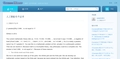# 人工智慧設計必讀

[1 paradox]Why 0.999... is not equal to 1?
Written in 2012
The current mathematic theory tells us, 1>0.9, 1>0.99, 1>0.999, ..., but at last it says 1=0.999..., a negation of itself (Proof 0.999... =1: 1/9=0.111..., 1/9x9=1, 0.111...x9=0.999..., so 1=0.999...). So it is totally a paradox, name it as 【1 paradox】.
......

### 回應http://courseminer.com/question/113You see this is a mathematic problem at first, actually it is a philosophic problem. Then we can resolve it. Because math is a incomplete theory, only philosophy could be a complete one. The answer is that 0.999... is not equal to 1. Because of these reasons:

1. The infinite world and finite world.
......